\$B:#>,\$N0lGU(B

\$BKhF|!":#>,\$N0lGU\$r>e\$2\$F\$\$\$^\$9!#(B
\$B:#>,0{\$`\$b\$N\$K:\$\$C\$?\$i!";29M\$K\$7\$F\$_\$F\$/\$@\$5\$\$!#(B

2021\$BG/(B4\$B7n(B

 1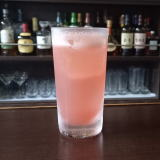(IN0L_(B 2(I2><^](B 3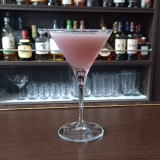(IO7^0%L^Y0(B 4 \$B5Y\$_(B 5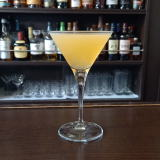(IL_[KK^<.](B 6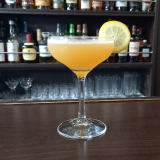(IL_X]=%A,0Y=^(B 7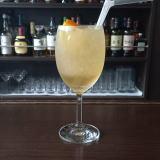(I<*X0%:L^W0(B 8 \$B5Y\$_(B 9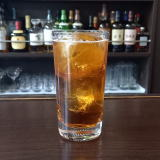(IY0<^-%J^/8(B 10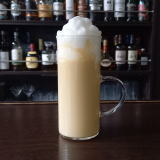(IN/D%4/8^%I/8^(B 11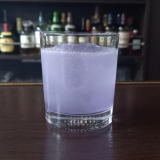(I>V](B 12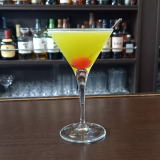(IL_W3D^%O](B 13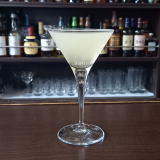(IL_X]=%4D^\0D^%OC(0(B 14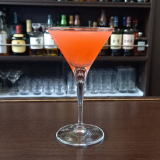(IL_XOD^]E(B 15 \$B5Y\$_(B 16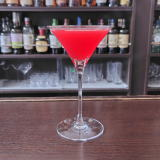(IY0=^M^YD(B 17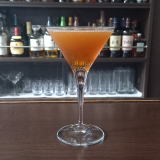(I[0Y=[2=(B 18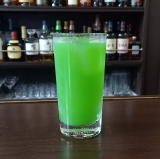(IF]<^,%@0DY(B 19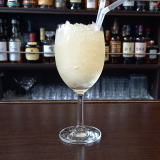(I<*X0%;]6^X0(B 20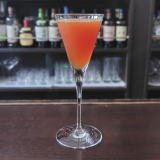(IA-0X/L_(B 21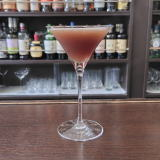(I1]@/A,L^Y(B 22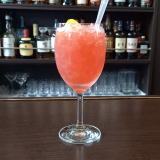(IC70W%C^2<^0(B 23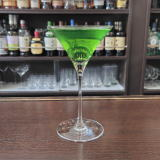(I>]DJ_DX/8=%C^0(B 24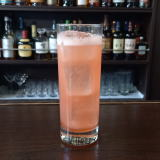(I=J_F/<-%12=^(B 25 \$B5Y\$_(B 26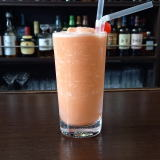(I4]<^*Y%=M_<,Y(B 27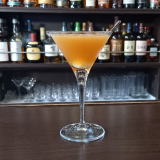(I24[0%W/DW0(B 28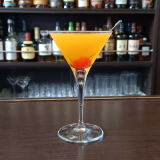(I;]8=7^K^]8^%\$B#s#p(B 29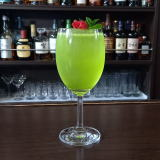(I32=^X0%6^0C^](B 30(I32=70%C^2<^0(B